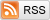# The LaTex Numbers

October 12, 2013

Let's define the LaTex numbers to be the set of all real numbers that can be unambiguously expressed with the LaTex type system. This set of numbers has a few fun properties, not least of which, as we'll see later, is that it doesn't quite exist.

Firstly, the LaTex numbers contain all rational numbers: to express a rational number in LaTex, you can just write it out. Furthermore, the LaTex numbers contain the larger set of all algebraic numbers, because you can write out any polynomial with integer coefficients in LaTex, and then say that you want its third largest root, for example. Even more generally, the LaTex numbers contain all computable numbers, ie, numbers that can be computed to arbitrary precision with a finite algorithm in finite time, because you can write out that algorithm in LaTex. Finally, every specific number that anyone has ever uttered or written about is a LaTex number: just write out whatever that person said or wrote about in LaTex, (using UTF-8 if necessary!)

Despite how large this set is, the set of LaTex numbers is still countable, since LaTex source code is ultimately a long (but finite) string of bits. This string of bits is a large integer expressed in binary, and there are only countably many integers, and so countably many LaTex numbers. This means that almost all numbers are not LaTex numbers, (since any countable set of real numbers has Lebesgue measure zero).

So what's an example of a non-LaTex number? At first, I thought the following: Well, we know that a whole lot of these numbers exist, since there are uncountably many of them. We know in fact that almost every number is not a LaTex number. But we can't actually know any specific non-LaTex numbers--if I could describe one to you, then I could write up that description in LaTex, and then it wouldn't be a LaTex number!

But then, my friend David Chudzicki pointed out the following interesting diagonalization argument: Take all of the LaTex numbers and enumerate them. This is easy enough to do unambiguously, at least in theory: Each LaTex number has many different representations in LaTex, each of which, as I pointed out earlier, is ultimately just a long integer in binary. For each LaTex number then, simply pick the smallest possible one of these binary integers, and sort the LaTex numbers by these quantities. (This amounts to identifying each LaTex number with the alphabetically first LaTex document that describes it, and then sorting the LaTex numbers by these identifying documents).

After unambiguously enumerating the LaTex numbers, we take the LaTex numbers that are in [0, 1], and write them out in binary, (actual binary this time, not the binary representation of a LaTex document. So, for example, the LaTex number "one third" becomes .0101010101...) We then write out the strings of numbers on top of each other, in order. (In the case of the dyadic rationals in (0, 1), which have two different representations, like "one half" = 0.1000000... = 0.0111111..., we write out both in lexicographical order). We then take the number that is the bitwise negation of the diagonal. So if, for example, the first few strings were

```.1011...
.1101...
.0001...
.1110...
....
```

then the number that I'm describing, the bit-flip of the diagonal, would be .0011... By construction, this number cannot be a LaTex number, because if it were, then it would be in [0, 1], and so would have to appear as, say, the nth sequence in our enumeration. But this number can't be the nth sequence, because by construction it disagrees with the nth sequence at the nth bit.

So this special number that I explicitly described can't be a LaTex number. But it also must be! I just described it explicitly in html, and could easily write up this description in LaTex. What does this mean? Well, it means that the concept of a "LaTex number" is not actually well-formed. This is why mathematicians formally define this idea with the concept of the definable numbers.

PS: You might think that the Microsoft Word numbers are a strict subset of the LaTex numbers, but that is in fact not true. In Microsoft Word, you can always write: "The following is a hexadecimal representation of an ASCII-encoded LaTex document describing my number: 4e 65 76 65 72 20 67 6f 6e 6e 61 20 67 69 76 65 20 79 6f 75 20 75 70 0d 0a 1f 4e 65 76 65 72 20 67 6f 6e 6e 61 20 6c 65 74 20 79 6f 75 20 64 6f 77 6e..."